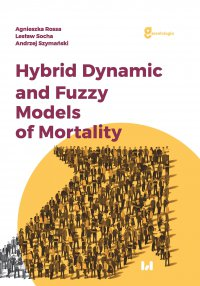# Hybrid Dynamic and Fuzzy Models of Morality

22,45 zł

Dostępne formaty plików: PDF

 Wydawnictwo Wydawnictwo Uniwersytetu Łódzkiego ISBN 978-83-8088-927-9 Data wydania 2018 Język: Angielski Liczba stron: 303 Rozmiar pliku: 4,2 MB Zabezpieczenie: Znak wodny

### Opis

The mortality modeling and forecasting is of fundamental importance in many areas, such as funding of public and private pensions, life insurance, the care of the elderly or the provision of health services. The book is an attempt to approach this subject from a new theoretical point of view, using theory of stochastic differential equations, theory of fuzzy numbers and complex numbers. These notes are addressed to tertiary students, doctoral students and specialists in the fields of demography, life insurance, statistics and economics.

### Spis treści

Preface 11

Abbreviation and notation 13

Introduction 15

Chapter 1. Basic mortality characteristics and models 19

1.1. Introduction 19

1.2. Discrete-time mortality frameworks 19

1.3. Continuous-time mortality frameworks 27

1.4. Laws of mortality 32

1.5. The Lee-Carter model and its extensions 36

1.6. The dynamic Lee-Carter model 49

1.7. The Vasicek and Cox-Ingersoll-Ross models 53

1.8. The Milevsky-Promislow model 59

1.9. The Giacometti-Ortobelli-Bertocchi model 63

1.10. The modified Milevsky-Promislow model 68

1.11. The Milevsky-Promislow models with two or more linear scalar filters 72

1.12. Final remarks 75

Chapter 2. Static and dynamic hybrid models 77

2.1. Introduction 77

2.2. Static hybrid models 78

2.3. Dynamic hybrid models 80

2.4. Moment equations for the hybrid models 86

2.5. Final remarks 92

Chapter 3. Dynamic hybrid mortality models 93

3.1. Introduction 93

3.2. Identification of switchings 95

3.3. The dynamic Lee-Carter hybrid model 108

3.4. The Vasicek and Cox-Ingersoll-Ross hybrid models 111

3.5. The Milevsky-Promislow hybrid models with one linear scalar filter 121

3.6. The Giacometti-Ortobelli-Bertocchi hybrid models 126

3.7. Modified Milevsky-Promislow hybrid models 131

3.8. The Milevsky-Promislow hybrid models with two or more linear filters 136

3.9. Final remarks 158

Chapter 4. Mortality model based on oriented fuzzy numbers 161

4.1. Introduction 161

4.2. Algebra of oriented fuzzy numbers 162

4.3. The extended Koissi-Shapiro mortality model 173

4.4. Data fuzzification with switchings 176

4.5. Parameters’ estimation of the EFLC model 180

4.6. Final remarks 182

Chapter 5. Mortality models based on modified fuzzy numbers and complex functions 183

5.1. Introduction 183

5.2. Mortality model based on the algebra of modified fuzzy numbers 183

5.3. Parameters’ estimation of the MFLC model 186

5.4. Mortality model based on complex functions 190

5.5. Parameters’ estimation of the CFLC model 193

5.6. Quaternion-valued mortality model 194

5.7. Parameters’ estimation of the QVLC model 199

5.8. Final remarks 202

Chapter 6. Models’ estimation and evaluation based on the real data 203

6.1. Introduction 203

6.2. Results of switching points’ identification for the mortality data of Poland 204

6.3. Estimation results 205

6.4. Final remarks 230

Appendix A. Elements of the analysis of stochastic processes and stochastic equations 231

A.1. Basic definitions of stochastic processes 231

A.2. Differential and integral calculus of stochastic processes 242

A.3. Moment equations for linear stochastic dynamic systems 253

A.4. Methods of discretization of stochastic differential equations 259

Appendix B. Elements of the algebra of modified fuzzy and complex numbers 261

B.1. Modified fuzzy numbers 261

B.2. Complex numbers and complex functions 268

Index 285

Bibliography 291

Korzystamy z plików cookies w celu sprawnej realizacji usług i poprawnego działania strony.
Możesz określić sposób przechowywania lub dostępu do plików cookies w Twojej przeglądarce. Więcej informacji znajdziesz tutaj »